# Fundamentals of image gradients and edge detectionImage gradient is nothing but directional change in image intensity. By computing gradient for a small area of image and repeating the process for entire image, we can detect edges in images. Edge detection is pervasive in several applications such as finger print matching , medical diagnosis and license plate detection. These applications basically highlights the areas where image intensity changes drastically and ignore everything else.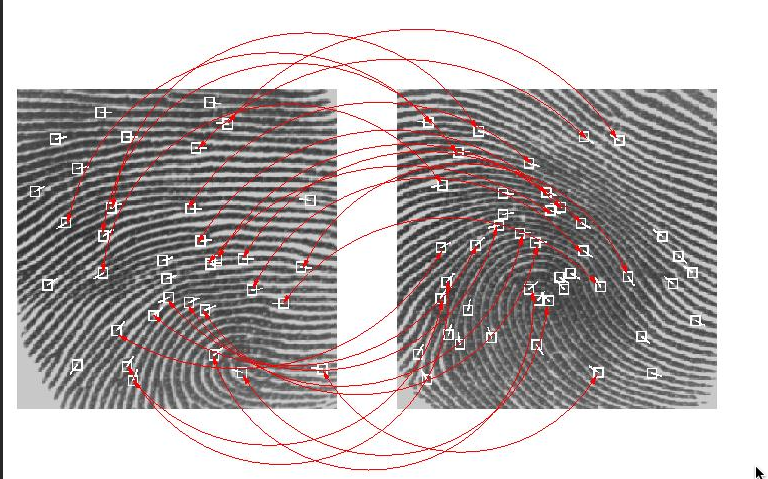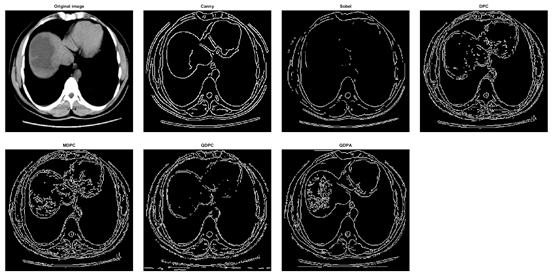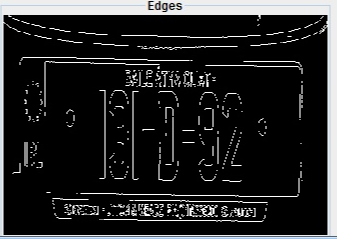Gradient computation involves computation of gradient magnitude and direction. To compute magnitude, we first need to compute gradient in x (Gx) and y(Gy) directions. Consider a rectangle image below. To compute gradient Gx, we move from left to right and highlight the points where image intensity is changing drastically. This would give us points along vertical edges of rectangle. Similarly to compute gradient Gy, we move from top to bottom and highlight the points where image intensity is changing drastically. This would give us points along horizontal edges of rectangle.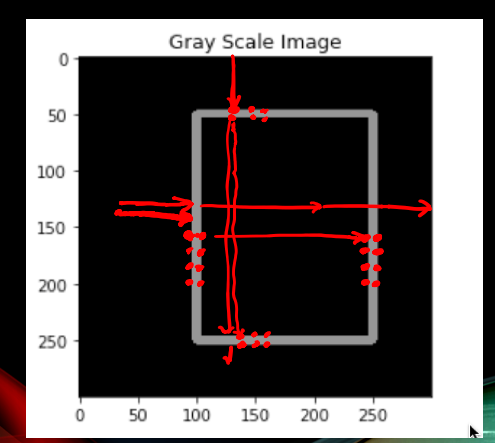The following image shows how Gx, Gy is computed first and then combined to give overall gradient magnitude.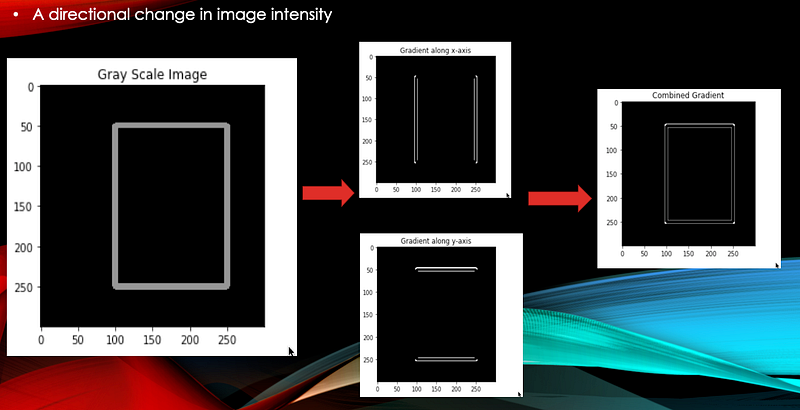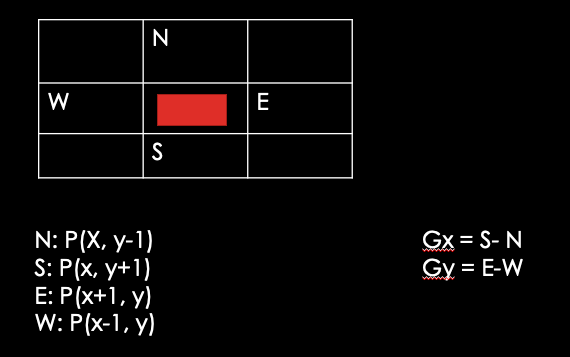As you can see in example above Gx is just difference between pixels intensity of points to east and west of center point. Similarly Gy is difference in pixel intensity of points to south and north of central pixel. Now that we have Gx and Gy, let’s see how can compute G.

Mathematically Gradient magnitude is given as

G = Sqrt (GX**2 + Gy**2)

and Gradient direction is given as

Theta = atan2(Gy,Gx) * 180/pi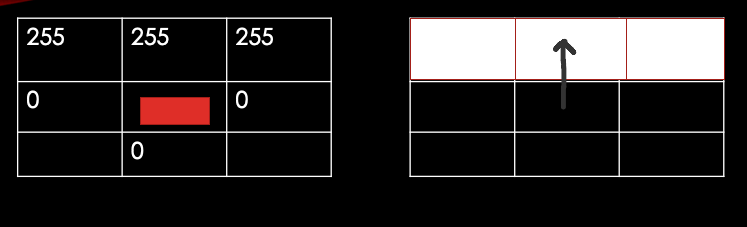In above example all changes are happening to north of central point. Here Gx =0 , Gy=-255. Hence Theta=-90 degrees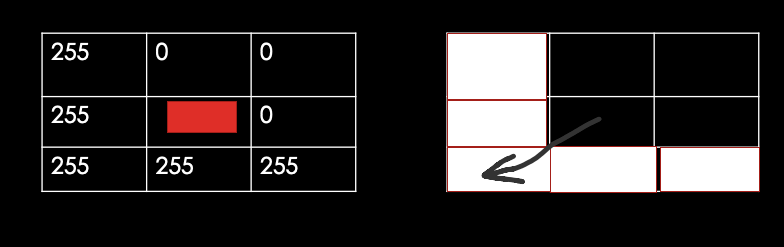In above example, Gx = 255, Gy = 255

G= Sqrt(255**2 + 255**2) = 255 and

Theta=atan2(255,255)*180/pi=135 degrees

This gives G and theta only for a small 3x3 area of whole image. You would then need to repeat the whole process for entire image.

Fortunately this can be achieved easily with convolution of predefined kernels. Lets discuss few here.

Sobel Kernels:

There are 2 predefined 3x3 sobel kernels Kx and Ky that are used to compute gradients. These kernels are applied to 3x3 area of image (I) at a time using convolution and then is repeated across the whole image. Sobel kernels gives more weightage to center prixels as compares to other pixels.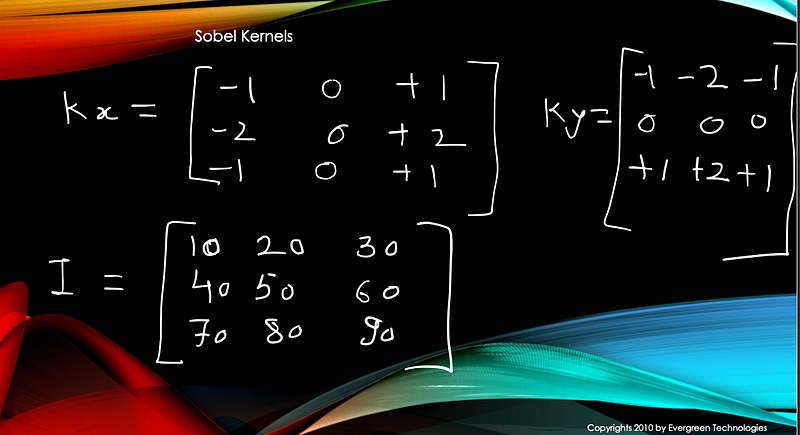Gx = I convolve Kx

Gy = I convolve Ky

Here convolve operation is element wise multiplication of matrix elements and then summing it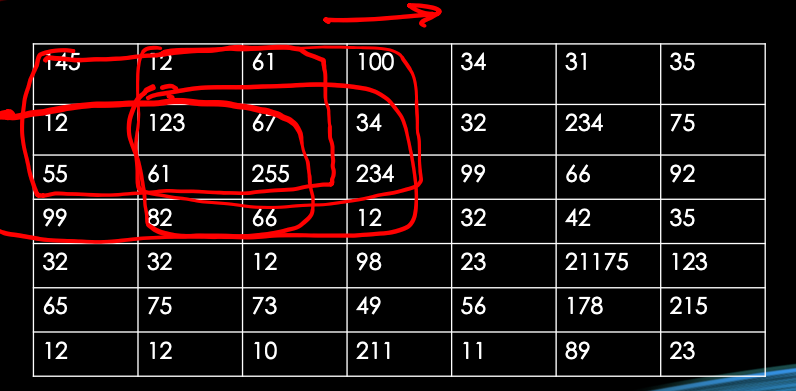We then repeat the process by sliding kernels from left to right and top to bottom.

This id handled behind the scenes for you if you use opencv and python. Here is code for computing Sobel gradient

# compute the gradient magnitude and orientation respectively # compute gradients along the X and Y axis, respectively gX = cv2.Sobel(gray, ddepth=cv2.CV_64F, dx=1, dy=0) gY = cv2.Sobel(gray, ddepth=cv2.CV_64F, dx=0, dy=1) mag = np.sqrt((gX ** 2) + (gY ** 2)) orientation = np.arctan2(gY, gX) * (180 / np.pi) % 180

cv2.imshow(“gradient magnitude”, mag)

Here is visual effect of Sobel Gradient. Notice how edges are being detected.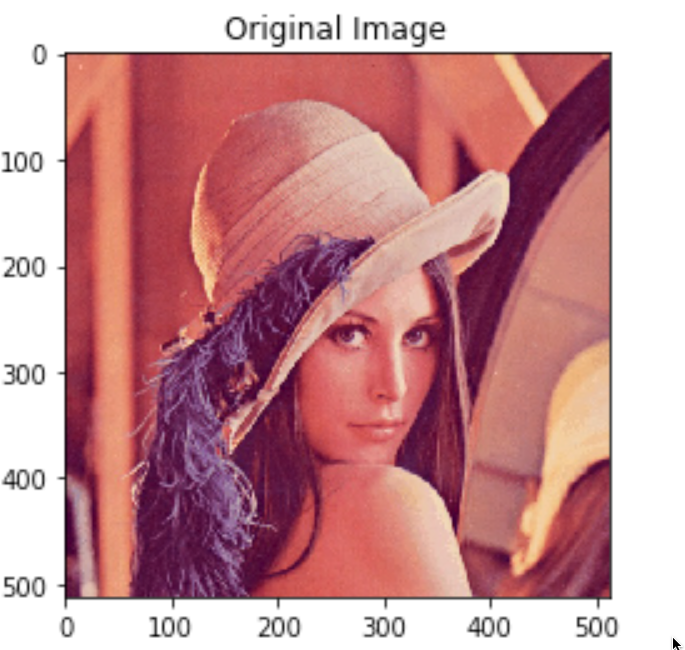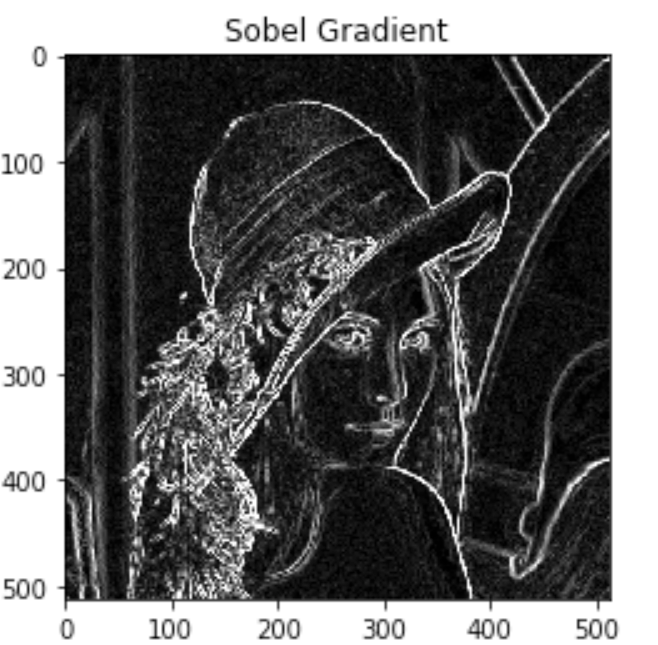Scharr:

Scharr kernels are given below. There are just another variant of Sobel kernels. It works better when kernel size is 3x3 as compares to Sobel filter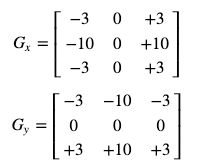Here is code

# compute the gradient magnitude and orientation respectively # compute gradients along the X and Y axis, respectively gX = cv2.Scharr(gray, ddepth=cv2.CV_64F, dx=1, dy=0) gY = cv2.Scharr(gray, ddepth=cv2.CV_64F, dx=0, dy=1) mag = np.sqrt((gX ** 2) + (gY ** 2)) orientation = np.arctan2(gY, gX) * (180 / np.pi) % 180

cv2.imshow(“gradient maginitude”, mag)

And here is visual representation of Scharr kernel gradient magnitude.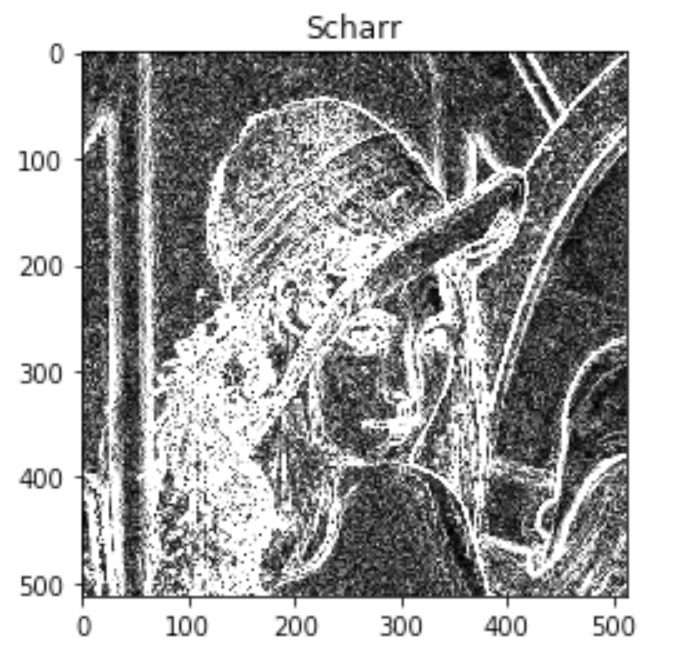Scharr and Sobel are first order derivatives.

Laplacian:

Laplacian is second order derivative used for detecting edges.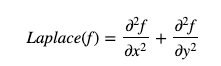Here is code or laplacian edge detection

laplacian = cv2.Laplacian(blur_img, cv2.CV_64F)

cv2.ishow(laplacian)

Ansd here is the output.Note that edges are very subtle.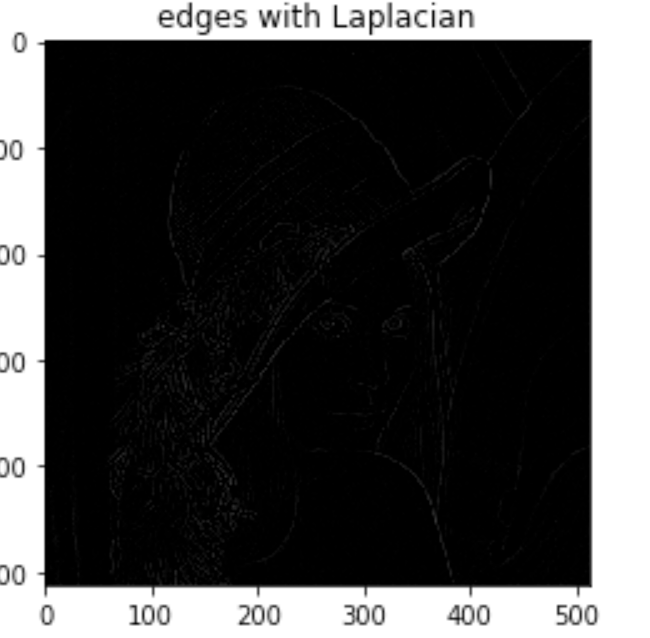Canny:

Canny is one the most popular edge detection technique. It is multi stage algorithm. It was developed by John F Canny in 1986.

Here are the steps

•Apply Gaussian Blur : Removes noise in the image

•Compute Gx, Gy using Sobel : Applies sobel kernels

•Apply nonmaxima suppression : Thins edges by removing non-maxima points

•Hystersis thresholding : Removes points which are not on edges

Here is the code

edges = cv2.Canny(gray, 100, 200, 3, L2gradient=True)

cv2.imshow(“canny”, edges)

and here is the Canny edge detector output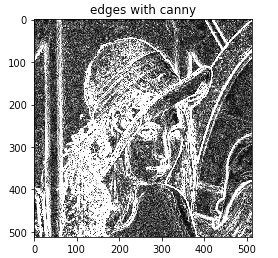Applying to gradients to video in real time :

Videos are nothing but series of images. So I attempted to apply these edge detection algorithms to video captured from my webcam. You can see the output here and compare the performance of each edge detection algorithm.

If you would like to learn step by step on how to do edge detection, you can sign up for my course here. No background in opencv is assumed. Basic image math and basic convolution and gradient operations are illustrated with several code examples. Email me at evergreenllc2020@gmail.com for discounts.

About Author Evergreen Technologies:

Active in teaching online courses in Computer vision , Natural Language Processing and SaaS system developmentOver 20 years of experience in fortune 500 companies

•Linked in: @evergreenllc2020

•Twitter: @tech_evergreen

•Udemy: Evergreen Technologies

Over 10,000 students in 145 countries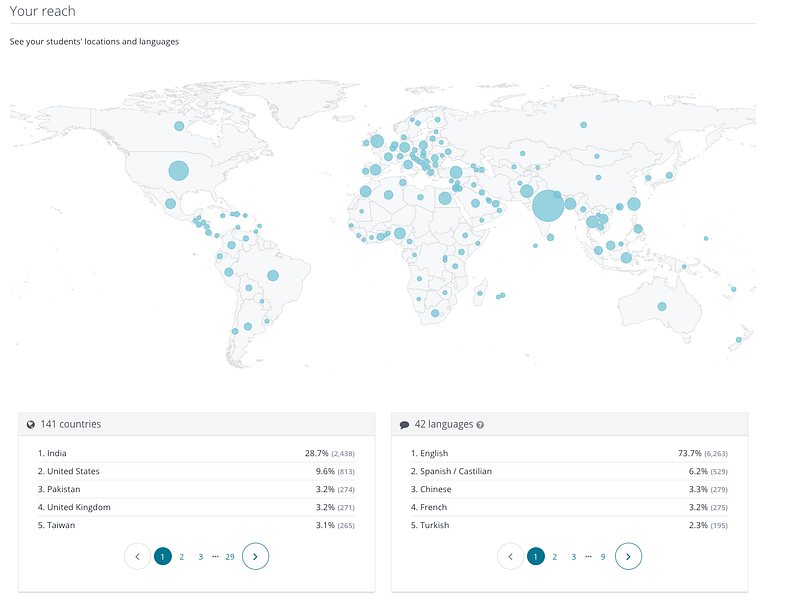See All